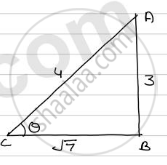Share
Notifications

View all notifications
Books Shortlist
Your shortlist is empty

# If Sin Theta = 3/4 Prove that Sqrt(Cosec^2 Theta - Cot)/(Sec^2 Theta - 1) = Sqrt7/3 - CBSE Class 10 - Mathematics

Login
Create free account

Forgot password?

#### Question

if sin theta = 3/4  prove that sqrt(cosec^2 theta - cot)/(sec^2 theta - 1) = sqrt7/3

#### Solution

We have sin theta = 3/4In ΔABC

AC^2 = AB^2 + BC^2

=> (4)^2 = (3)^2 + BC^2

=> BC^2= 16 - 9

=> BC^2 = 7

=> BC = sqrt7

:. cosec theta = 4/3, sec theta = 4/sqrt7 and cot theta = sqrt7/3

Now

L.H.S sqrt((cosec^2 theta - cot^2 theta)/(sec^2 theta - 1))

= sqrt(((4/3)^2 - (sqrt7/3)^2)/((4/sqrt7)^2 - 1)

= sqrt((16/9 - 7/9)/(16/7 - 1)

=sqrt((9/9)/((16 - 7)/7 ))

= sqrt(7/9)

= sqrt7/3

= R.H.S

Is there an error in this question or solution?

#### APPEARS IN

Solution If Sin Theta = 3/4 Prove that Sqrt(Cosec^2 Theta - Cot)/(Sec^2 Theta - 1) = Sqrt7/3 Concept: Trigonometric Ratios.
S